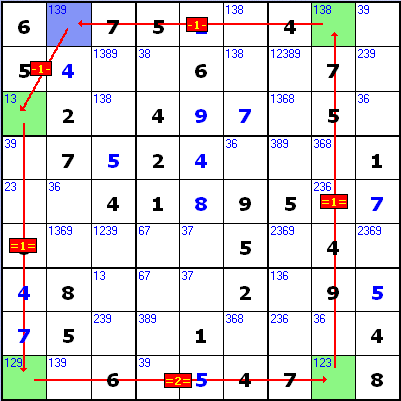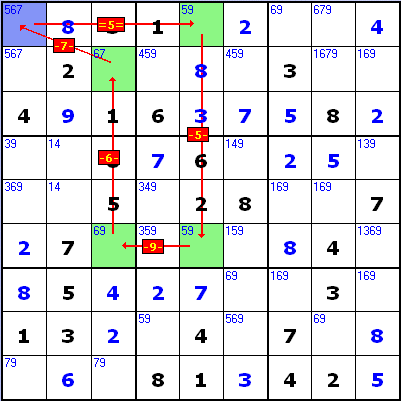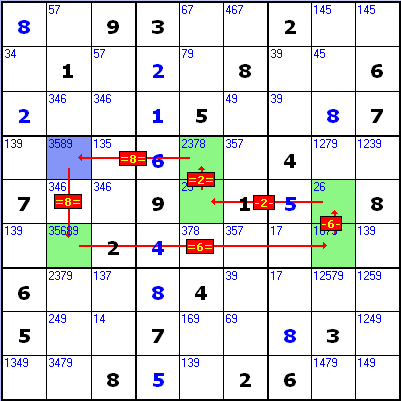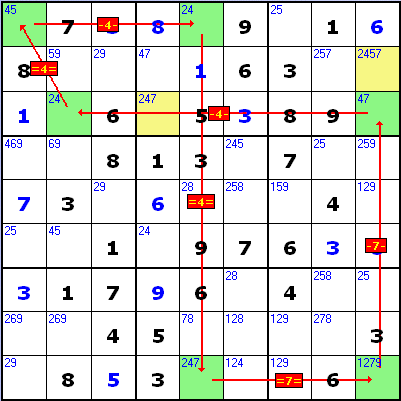Mirrored from Sudopedia, the Free Sudoku Reference Guide

# Nice Loop

A Nice Loop is a loop that follows a set of rules and notation guidelines. Several types of chains and loops can also be represented as Nice Loops.

The basis for a Nice Loop is the B/B Plot, which shows all available strong links in the grid. The nodes in a Nice Loop are cells, not individual candidates as in Alternating Inference Chains. The links between candidates in a single cell are implied. The connections between the cells tell us how the candidates inside are interacting.

Each Nice Loop is a Double Implication Chain, where implications are propagated in both directions.

## Nice Loop terminology

Please make sure that you understand the following terms, which are explained separately in Sudopedia.

There must be a link between each pair of nodes in a Nice Loop. For two nodes to be linked, they must be peers. The type of link plays an important role. A strong link can be used for strong or weak inference, but a weak link can only be used for weak inference. Hence, there are 3 types of links that can exist between 2 nodes:

• A strong link with strong inference
• A strong link with weak inference
• A weak link with weak inference

The last two are equivalent in Nice Loops.

## Propagation Rules

The strict Nice Loop propagation rules ensure that all nodes are properly connected. A loop is not a Nice Loop when it does not comply with these rules.

• When a node has 2 links with strong inference, the digits must be different.
• When a node has 2 links with weak inference, the cell must be bivalue and the digits must be different.
• When a node has 2 links with different inference, the digits must be the same.

A chain can only be a loop when it starts and ends with the same cell. This cell forms the first and the last node in the chain. These are separate nodes, each with a single link. Both links do not need to comply with the propagation rules.

## Continuous and Discontinuous Nice Loops

To determine whether a Nice Loop is continuous or discontinuous, we must examine the first and the last node, representing the same cell. When both links to that cell comply with the propagation rules, the loop is continuous, otherwise it is discontinuous.

### Continuous Nice Loops

These are rare, but very effective. For every node cell having 2 links with strong inference, all candidates in the cell which are not used in the links can be eliminated. All remaining candidates in the houses that provide the weak links can also be eliminated.

### Discontinuous Nice Loops

In a discontinuous loop, the first/last cell is referred to as the discontinuity. Discontinuous loops are more frequent, but they only have an effect on the discontinuity. This effect depends on the type of links it has to the adjacent nodes.

• When the discontinuity has 2 links with weak inference for a single digit, this digit can be eliminated from the discontinuity.
• When the discontinuity has 2 links with strong inference for a single digit, this digit can be placed in the discontinuity.
• When the discontinuity has 2 links with different inference for 2 different digits, the digit providing the weak inference can be eliminated from the discontinuity.

## Nice Loop Notation

Nice loops are written as chains. The chain always starts and ends with the same cell. In a discontinuous loop, this must be the discontinuity. The cell names are enclosed in square brackets. These names are preferably written in rncn notation.

```[r1c1]
```

Links with strong inference are represented by =X=, where X is the digit. Links with weak inference are represented by -X-. To make a distinction between continuous and discontinuous loops, a single dash or equal sign is placed at the start and end of the loop to indicate how it continues.

```=[r1c1]-1-[r4c1]=1=[r4c5]-1-[r1c5]=1=[r1c1]-
```

These continuation markers are not present in discontinuous loops.

```[r1c1]-1-[r4c1]=1=[r4c5]-1-[r5c6]=1=[r1c6]-1-[r1c1]
```

## Examples

This example shows a discontinuous nice loop, where the discontinuity has weak inference:```[r1c2]-1-[r3c1]=1=[r9c1]=2=[r9c8]=1=[r1c8]-1-[r1c2] => r1c2<>1
```

This example shows a discontinuous nice loop, where the discontinuity has different inference:```[r1c1]=5=[r1c5]-5-[r6c5]-9-[r6c3]-6-[r2c3]-7-[r1c1] => r1c1<>7
```

This example shows a discontinuous nice loop, where the discontinuity has strong inference:```[r4c2]=8=[r6c2]=6=[r6c8]-6-[r5c8]-2-[r5c5]=2=[r4c5]=8=[r4c2] => r4c2=8
```

This example shows a continuous nice loop (note - the loop's squares are green):```-[r1c5]=4=[r9c5]=7=[r9c9]-7-[r3c9]-4-[r3c2]=4=[r1c1]-4-[r1c5]= => r2c9<>7, r3c4<>4, r9c5<>2
```